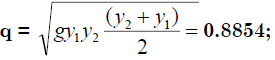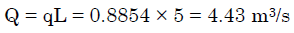Courses

# Compressible Flow And Flow Through Open Channel - MCQ Test 1

## 20 Questions MCQ Test Mock Test Series - Mechanical Engineering (ME) for GATE 2020 | Compressible Flow And Flow Through Open Channel - MCQ Test 1

Description
This mock test of Compressible Flow And Flow Through Open Channel - MCQ Test 1 for Mechanical Engineering helps you for every Mechanical Engineering entrance exam. This contains 20 Multiple Choice Questions for Mechanical Engineering Compressible Flow And Flow Through Open Channel - MCQ Test 1 (mcq) to study with solutions a complete question bank. The solved questions answers in this Compressible Flow And Flow Through Open Channel - MCQ Test 1 quiz give you a good mix of easy questions and tough questions. Mechanical Engineering students definitely take this Compressible Flow And Flow Through Open Channel - MCQ Test 1 exercise for a better result in the exam. You can find other Compressible Flow And Flow Through Open Channel - MCQ Test 1 extra questions, long questions & short questions for Mechanical Engineering on EduRev as well by searching above.
QUESTION: 1

Solution:

Ans. (c)

QUESTION: 2

Solution:
QUESTION: 3

### Acoustic velocity in an elastic gaseous medium is proportional to:

Solution:

Ans. (c)

QUESTION: 4

Assertion (A): In a supersonic nozzle, with sonic condition at the throat, any reduction of down stream pressure will not be felt at the inlet of the nozzle.
Reason (R): The disturbance caused downstream of supersonic flowstravels at sonic velocity which cannot propagate upstream by Machcone.

Solution:

Ans. (a)

QUESTION: 5

Consider the following statements pertaining to isentropic flow:
1. To obtain stagnation enthalpy, the flow need not be decelerate disentropically but should be decelerated adiabatically.
2. The effect of friction in an adiabatic flow is to reduce the stagnation pressure and increase entropy.
3. A constant area tube with rough surfaces can be used as a subsonicnozzle.
Of these correct statements are:

Solution:

Ans. (d) To obtain stagnation enthalpy, the flow must be decelerated isentropically.

QUESTION: 6

The Mach number at inlet of gas turbine diffuser is 0.3. The shape of the diffuser would be:

Solution:

Ans. (b)

QUESTION: 7

Consider the following statements:
1. De Laval nozzle is a subsonic nozzle.
2. Supersonic nozzle is a converging passage.
3. Subsonic diffuser is a diverging passage.
Which of these statements is/are correct?

Solution:

(d) Only third statement is correct, i.e. subsonic diffuser is a diverging passage.

QUESTION: 8

Consider the following statements:
A convergent-divergent nozzle is said to be choked when
1. Critical pressure is attained at the throat.
2. Velocity at the throat becomes sonic.
3. Exit velocity becomes supersonic.
Of these correct statements are

Solution:

Ans. (b) A convergent divergent nozzle is said to be choked when critical pressure
is attained at the throat and velocity at the throat becomes sonic.

QUESTION: 9

Consider the following statements :
Choked flow through a nozzle means:
1. Discharge is maximum
2. Discharge is zero
3. Velocity at throat is supersonic
4. Nozzle exit pressure is less than or equal to critical pressure.
Which of the above statements is/are correct?

Solution:

Ans. (d)

QUESTION: 10

Consider the following statements in relation to a convergent divergent steam nozzle operating under choked conditions:
1. In the convergent portion steam velocity is less than sonic velocity
2. In the convergent portion steam velocity is greater than sonicvelocity
3. In the divergent portion the steam velocity is less them sonicvelocity
4. In the divergent portion the steam velocity is greater than sonicvelocity
Which of the above statements are correct?

Solution:

Ans. (b)

QUESTION: 11

Which one of the following is correct?
For incompressible flow a diverging section acts as a diffuser for upstream flow which is:

Solution:

Ans. (c) For incompressible flow a diverging section acts as a diffuser for both
subsonic and supersonic. For compressible flow a diverging section acts as a diffuser for subsonic flow only.

QUESTION: 12

In turbulent flow over an impervious solid wall

Solution:

Ans. (d)

QUESTION: 13

Match List-I (Flow depth) with List-II (Basic hydraulic condition associated there with) and select the correct answer: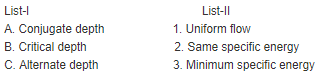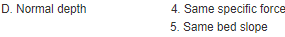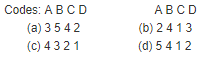Solution:

Ans. (c) Only one matching (B with 3) will give us ans. (c) The depth of flow at which specific energy is minimum is called critical depth.

QUESTION: 14

How is the best hydraulic channel cross-section defined?

Solution:

Ans. (c)

QUESTION: 15

Assertion (A): To have maximum hydraulic efficiency, the trapezoidal section of an open channel should be a half-hexagon
Reason (R): For any cross-section, a hexagon has the lest-perimeter.

Solution:

Ans. (c) We all knows that for any cross-section, a circular section has the lestperimeter.
So R is false.

QUESTION: 16

A hydraulic jump occurs in a channel

Solution:

Ans. (a) If the flow changes from supercritical to sub-critical. The hydraulic jump is defined as the sudden and turbulent passage of water from a supercritical state.

QUESTION: 17

Consider the following statements:
A hydraulic jump occurs in an open channel
1. When the Froude number is equal to or less than one.
2. At the toe of a spillway.
3. Downstream of a sluice gate in a canal.
4. When the bed slope suddenly changes.
Which of these are correct?

Solution:

Ans. (c) Only 1 is wrong so (a), (b) and (d) out.

QUESTION: 18

A sluice gate discharges water into a horizontal rectangular channel with a velocity of 12 m/s and depth of flow of 1 m. What is the depth offlow after the hydraulic jump?

Solution:

Ans. (c)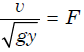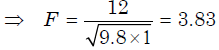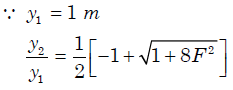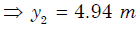QUESTION: 19

An open channel flow encounters a hydraulic jump as shown in the figure. The following fluid flow conditions are observed between A andB:
1. Critical depth
The correct sequence of the flow conditions in the direction of flow is: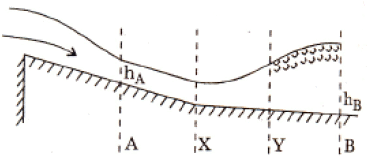Solution:

Ans. (b)

QUESTION: 20

A hydraulic jump is formed in a 5.0 m wide rectangular channel with sequent depths of 0.2 m and 0.8 m. The discharge in the channel, inm3/s, is:

Solution:

Ans. (c)